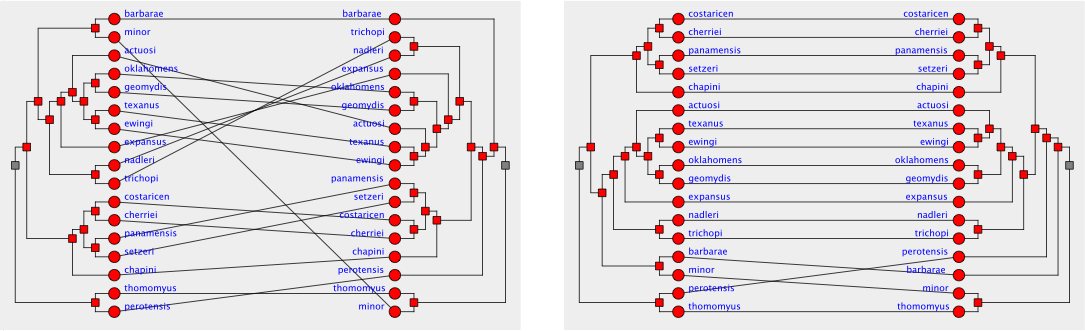I'm now in New York for ALENEX (today) and SODA (the following three days). The most interesting juxtaposition at ALENEX, for me, was the pair of back-to-back talks by Martin Nöllenburg and Siamak Tazari.

Martin spoke first, on binary tanglegrams. These are graph drawings in which one has two binary trees with the same set of leaf labels (say, two different hypotheses for the evolutionary tree on a given set of species); one draws the leaves of the trees in two side-by-side columns, with the trees extending outwards to the left and the right and with a bunch of edges in the middle connecting corresponding leaves. If the trees are really different, the middle edges form an unintelligible tangle of crossings (hence the name) but if the trees are similar then few crossings will be needed. However, one has to be careful in choosing the ordering of the children of each node in each tree, in order to find a permutation that results in few crossings. Here's figure 1 of Martin's paper showing two different layouts for a phylogenetic comparison of pocket gopher louse species (really, 17 different kinds of pocket gopher lice!): The right layout should be much more readable than the left.Martin had previously written a paper with half a dozen co-authors at Graph Drawing 2008 on approximation algorithms for permuting tanglegrams to minimize the number of crossings, and the research he presented today started out as an attempt to compare these methods experimentally with some other known methods. In the process of doing this testing he implemented a branch-and-bound exponential-time backtracking search algorithm for finding the optimal permutation of the two trees, and in order to make this algorithm work better he implemented a greedy algorithm for choosing which branch of the search tree to follow first. At which point he had a new heuristic for drawing tanglegrams: use the greedy algorithm and don't bother with the rest of the search. The results from his experiments were (disappointingly to the coauthors of the previous papers on the subject, no doubt) that the greedy method beats the approximation algorithms hands-down, despite not having a provable approximation bound. So, at least in this application, provable approximation ratio guarantees aren't necessarily much use in practice.

There's some interesting research remaining to be done here, by the way. Martin's implementation of the greedy heuristic takes quadratic time; is that necessary or can it be sped up? Is anything provable about its approximation ratio? What kinds of inputs are difficult for the greedy heuristic to optimize?

The second talk of the juxtaposition was the one by Siamak Tazari (with Matthias Müller-Hannemann), entitled Dealing with Large Hidden Constants: Engineering a Planar Steiner Tree PTAS. The problem here is one of finding, in a planar graph, a short tree that connects a given subset of terminal vertices. Cora Borradaile, Phil Klein, and Claire Mathieu (SODA '07 and WADS '07) had previously described an algorithm for approximating the optimal solution to this problem to within an approximation ratio of $$1+\epsilon$$, in time $$O(2^{O(\epsilon^{-9})}n+n\log n)$$, with the $$9$$ in the exponent of the exponent making this the epitome of an unimplementable theory-only algorithm. But Siamak showed that, with a few careful choices of some of the parameters of the algorithm (less conservative than the choices leading to a provable approximation ratio) one could implement an algorithm along the same lines that worked pretty well.

It's tempting to look for some theory to explain under what circumstances provable approximation algorithms work well in practice, and under what circumstances they don't. But maybe the better lesson to be drawn is the one Michael Mitzenmacher's been pushing for some time now: it's all very well to do theory, but if you want to claim your algorithm is practical you should back it up with experiments. In that sense, both of these papers are successes: one of them attempted to justify the practicality of a previous algorithm, and ended up discovering another even-better algorithm, and the second showed that an algorithm that looked likely to be useful only in theory could actually be made practical.